# How to Use HDL Optimized Normalized Reciprocal

This example shows how and when to use the `normalizedReciprocal` function and the Normalized Reciprocal HDL Optimized block to compute the normalized reciprocal of an input.

The reciprocal of a value can have a large range, and fixed-point types have limited range compared to floating-point types. If an input value `u` is small, then 1/u is large, and if u is large, then 1/u is small. Therefore, a fixed-point type for 1/u must have high precision and large range which requires a large word length.

In applications where the range of a product of a reciprocal and another variable is known, then it is efficient to compute a normalized reciprocal, multiply it by the other variable, and then apply a shift to the final output. For example, this is the case in least-squares applications where a division by a pivot element is required in back-substitution.

### Calculating the Normalized Reciprocal

Given an input `u`, Normalized Reciprocal computes the normalized reciprocal, `y`, and exponent, `e`, such that

` (2.^e).*y = 1./u,`

and

` 0.5 < |y| <= 1.`

If `u = 0` and `u` is fixed-point or scaled-double, then `y = 2 - eps(y)`.

If `u = 0` and `u` is a floating-point type, then `y = inf`.

If `u~=0`, this function returns the equivalent of

``` [y,e] = log2(1./abs(double(u))) y(u<0) = -y(u<0)```

except that it is computed using only shifts and adds.

### Choose the MATLAB Function or the Simulink Block

For C code generation and system design, use the MATLAB function `normalizedReciprocal`. This function does not compute with latency. For simulation, compile the function into a MEX file for speed using `fiaccel`, `buildInstrumentedMex`, or `codegen`.

To generate optimized HDL code, use the `Normalized Reciprocal HDL Optimized` block. This block is optimized for high throughput and small area in HDL, and simulates with the same latency present in the generated HDL code.

The block and function produce identical numerical outputs.

### Compute the Normalized Reciprocal Using MATLAB

Compute the normalized reciprocal of a fixed-point input, `u`, then compare this value to the actual value of the reciprocal.

```u = fi([-pi,0.01,pi]) ```
```u = -3.1416 0.0100 3.1416 DataTypeMode: Fixed-point: binary point scaling Signedness: Signed WordLength: 16 FractionLength: 13 ```
```[y,e] = normalizedReciprocal(u) ```
```y = -0.6367 0.7806 0.6367 DataTypeMode: Fixed-point: binary point scaling Signedness: Signed WordLength: 16 FractionLength: 14 e = 1x3 int32 row vector -1 7 -1 ```
```computed_reciprocal = 2.^double(e).* double(y) ```
```computed_reciprocal = -0.3183 99.9141 0.3183 ```
```actual_reciprocal = 1./double(u) ```
```actual_reciprocal = -0.3183 99.9024 0.3183 ```

You can see that the normalized reciprocal and the actual reciprocal are close in value.

### Define Inputs for Simulink Model

Define a fixed-point input, `u`, to take the normalized reciprocal of using the Normalized Reciprocal HDL Optimized block.

```x = linspace(0.001,100,100); x = [fliplr(-x),x]; u = fi(x,1,18); ```

### Latency of the Normalized Reciprocal HDL Optimized block

The Normalized Reciprocal HDL Optimized block works by normalizing the input using a binary search, which has a latency of approximately log2 of the word length of the input, followed by a CORDIC reciprocal kernel, which has a latency approximately the same as the word length of the input.

The Normalized Reciprocal HDL Optimized block is always ready to accept data. After the initial latency, valid samples are output every sample. The latency in samples for a fixed-point input `u` is

` D = ceil(log2(u.WordLength)) + u.WordLength + 5`

You can use the function `normalizedReciprocalLatency`, included in this example, to compute the latency for inputs with fixed point, double, or single numeric types.

To align the input samples with the output of the Normalized Reciprocal HDL Optimized block, use the latency `D` in a delay.

```D = normalizedReciprocalLatency(u) ```
```D = 28 ```

### Run the Normalized Reciprocal Simulink Model

As a simple example where the range of a product of a reciprocal and another variable is known, compute the normalized reciprocal of a value and multiply it by itself, so the final product should be equal to one. Even though the input value and reciprocal have large ranges, the product of the value and its reciprocal have a known range.

Note that the product `u*y` can be computed in the same type as `u` because `0.5 < abs(y) <= 1`, and so the product does not grow larger in magnitude.

```model = 'NormalizedReciprocalModel'; open_system(model) out = sim(model); ```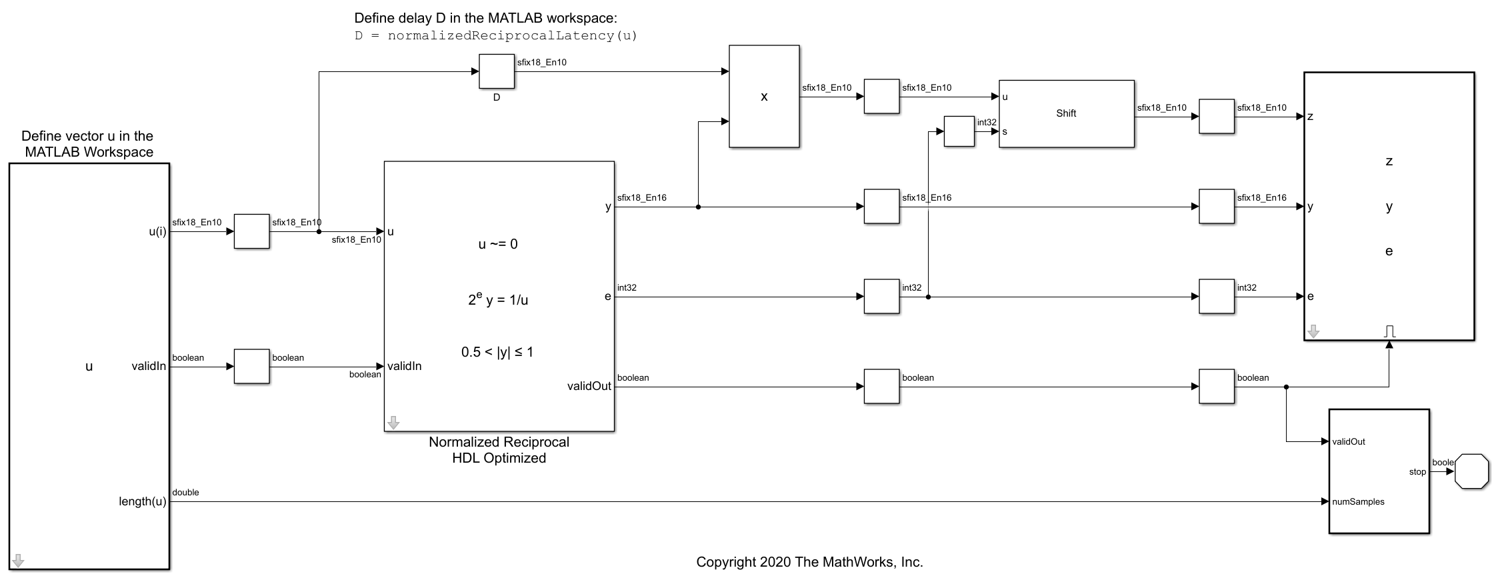### Analyze the Results of the Normalized Reciprocal Simulink Model

In the following plots, you can see that the input `u` and the reciprocal `1/u` have large ranges, but the normalized reciprocal `y` is in the range -1 to 1.

The input, `u`, is a vector of fi values which span the range of -100 to 100.

```normalizedReciprocalPlot(1,u,out.y,out.e,out.z); ```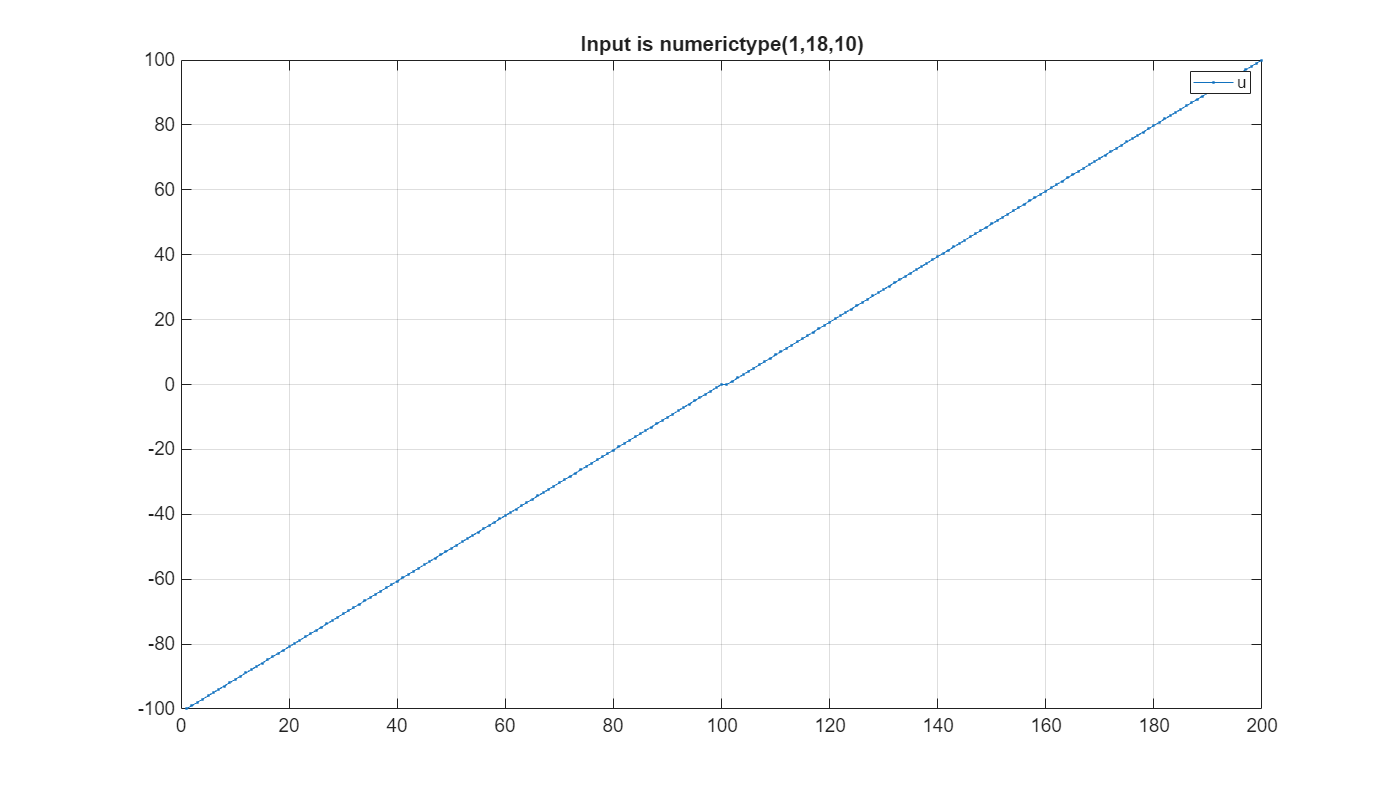The actual value of the reciprocal, `1/u`, is nearly identical to the normalized reciprocal, `(2.^e).*y`. The reciprocal `1/u` has a large range, which means that to compute a straight reciprocal in fixed point would require a data type with a high dynamic range (i.e. large word length and large fraction length).

```normalizedReciprocalPlot(2,u,out.y,out.e,out.z); ```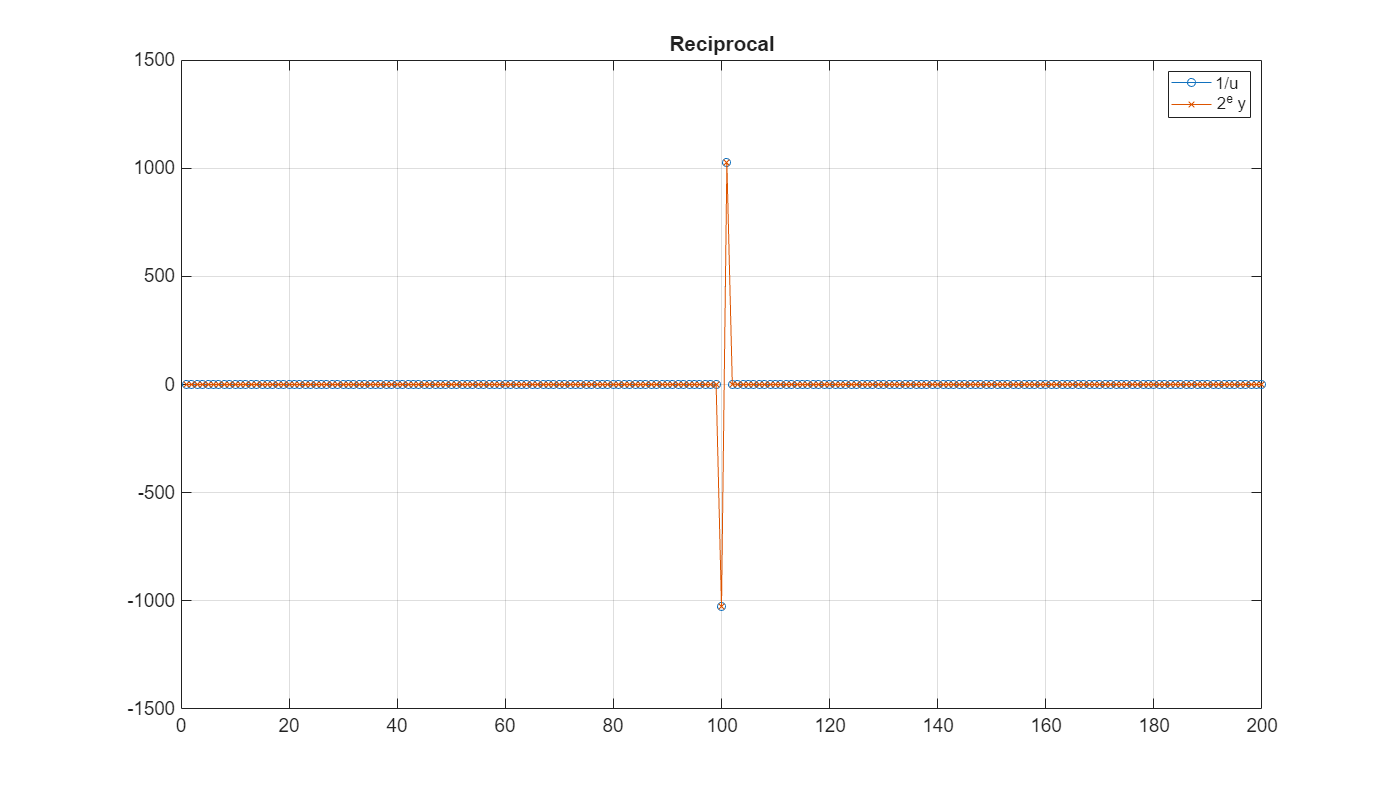The normalized reciprocal, `y`, is in the range

` 0.5 < |y| <= 1`

and can be efficiently and accurately stored in a data type with the same word length as `u`. The numeric type of `y` has a fraction length equal to two less than the word length of `u`. This additional integer bit ensures that `y` can be positive or negative, and can also exactly represent the values -1 and +1.

```normalizedReciprocalPlot(3,u,out.y,out.e,out.z); ```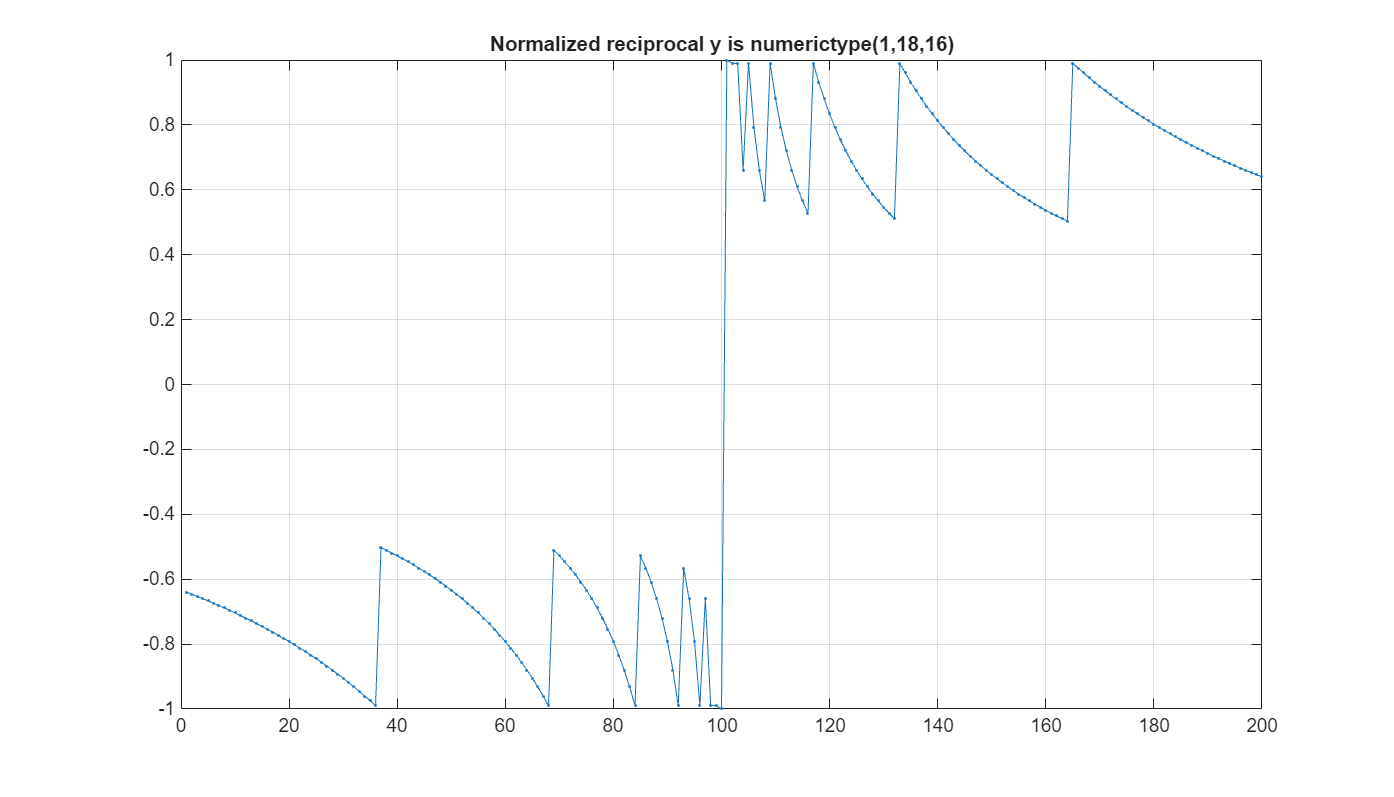The normalized exponent, `e`, and the magnitude of the input, `u`, have an inverse relationship. When `u` is large in magnitude, then `e` is a large negative value. When `u` is close to zero, then `e` is a large positive value. The relationship is

` (2.^e).*y = 1./u,`
```normalizedReciprocalPlot(4,u,out.y,out.e,out.z); ```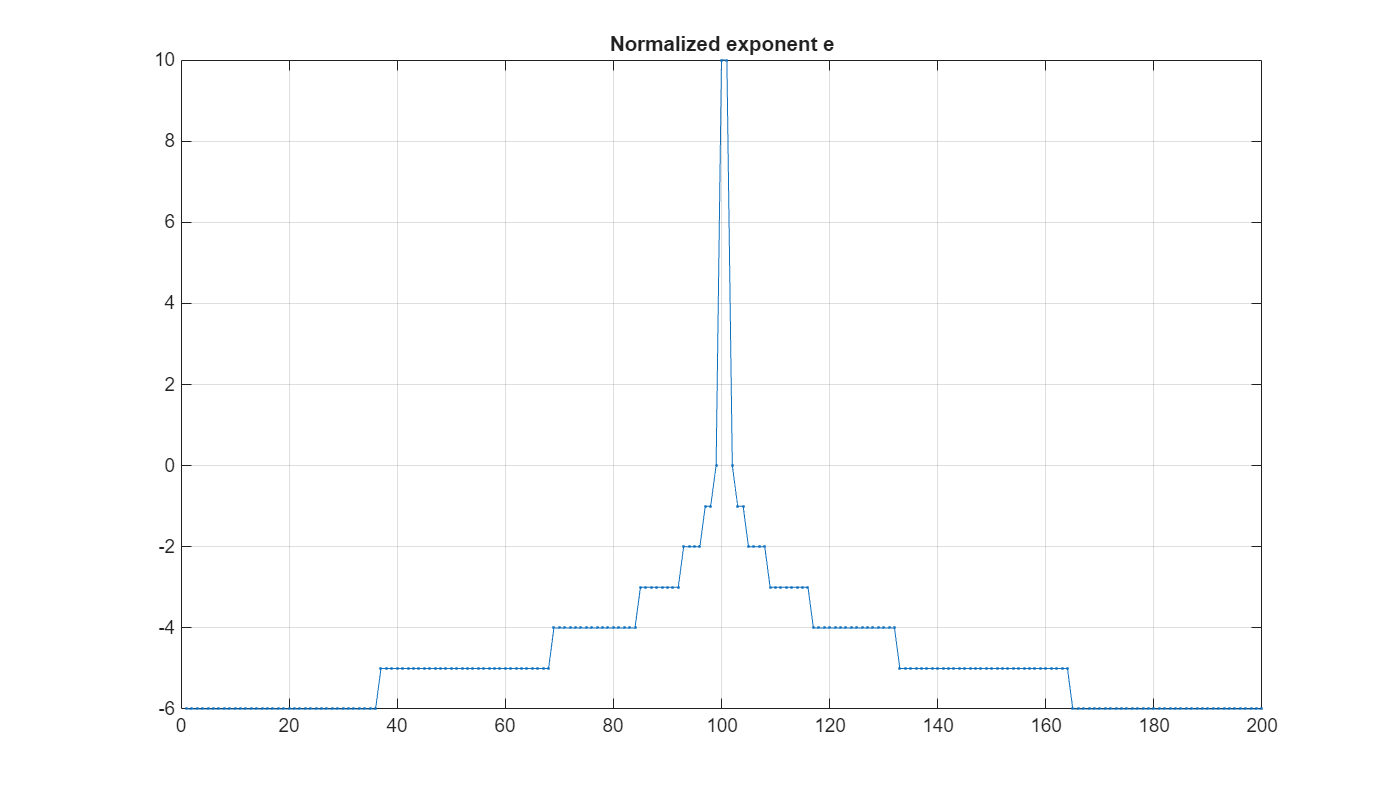The model multiplies the normalized reciprocal `y` by the input `u` and then scales the result by shifting by `e`. Since `y` has a magnitude less than 1, the product can be done in the fixed-point type of `u`. The output, z = 2^e (y * u), is approximately equal to 1.

```normalizedReciprocalPlot(5,u,out.y,out.e,out.z); ```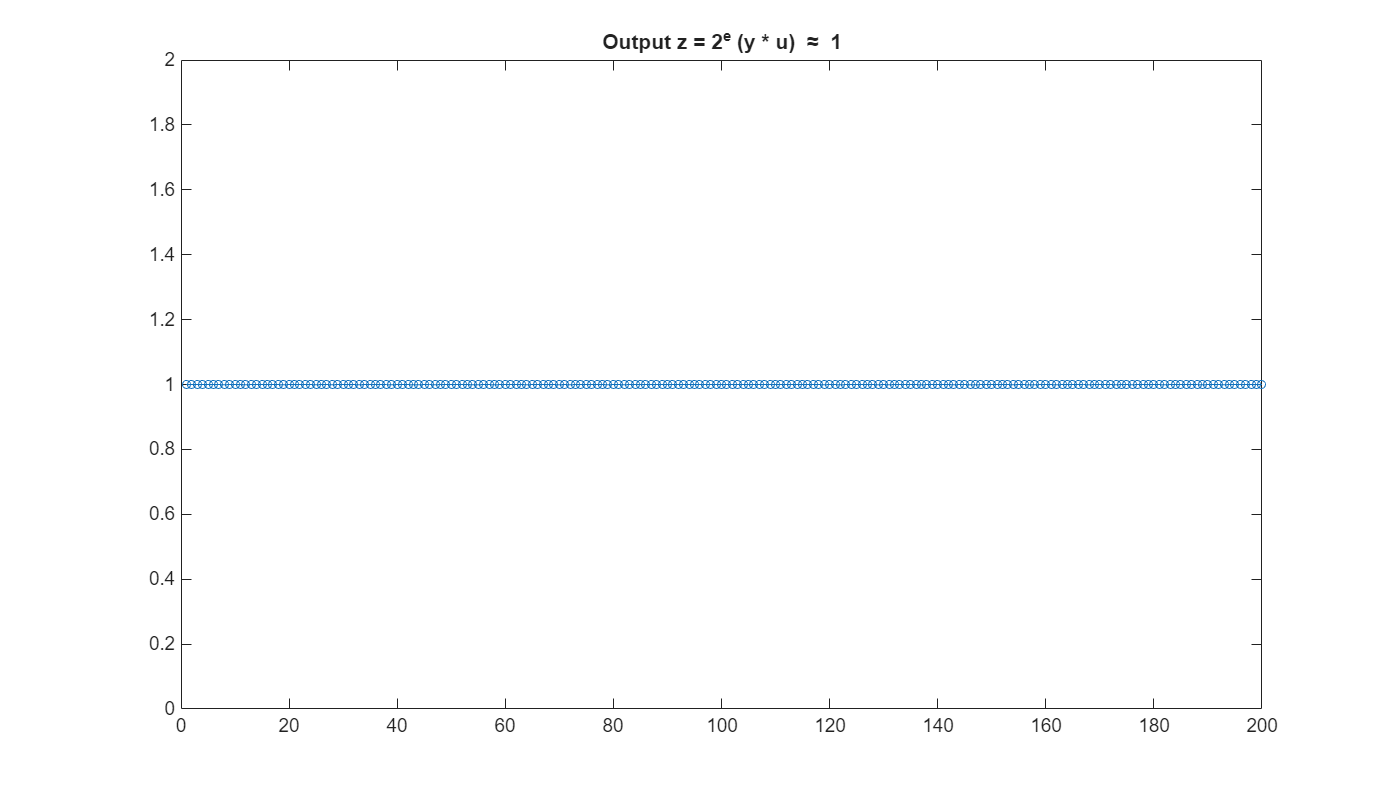The output, `z`, has a small roundoff error as a result of computing the normalized reciprocal with fixed-point data types.

```normalizedReciprocalPlot(6,u,out.y,out.e,out.z); ```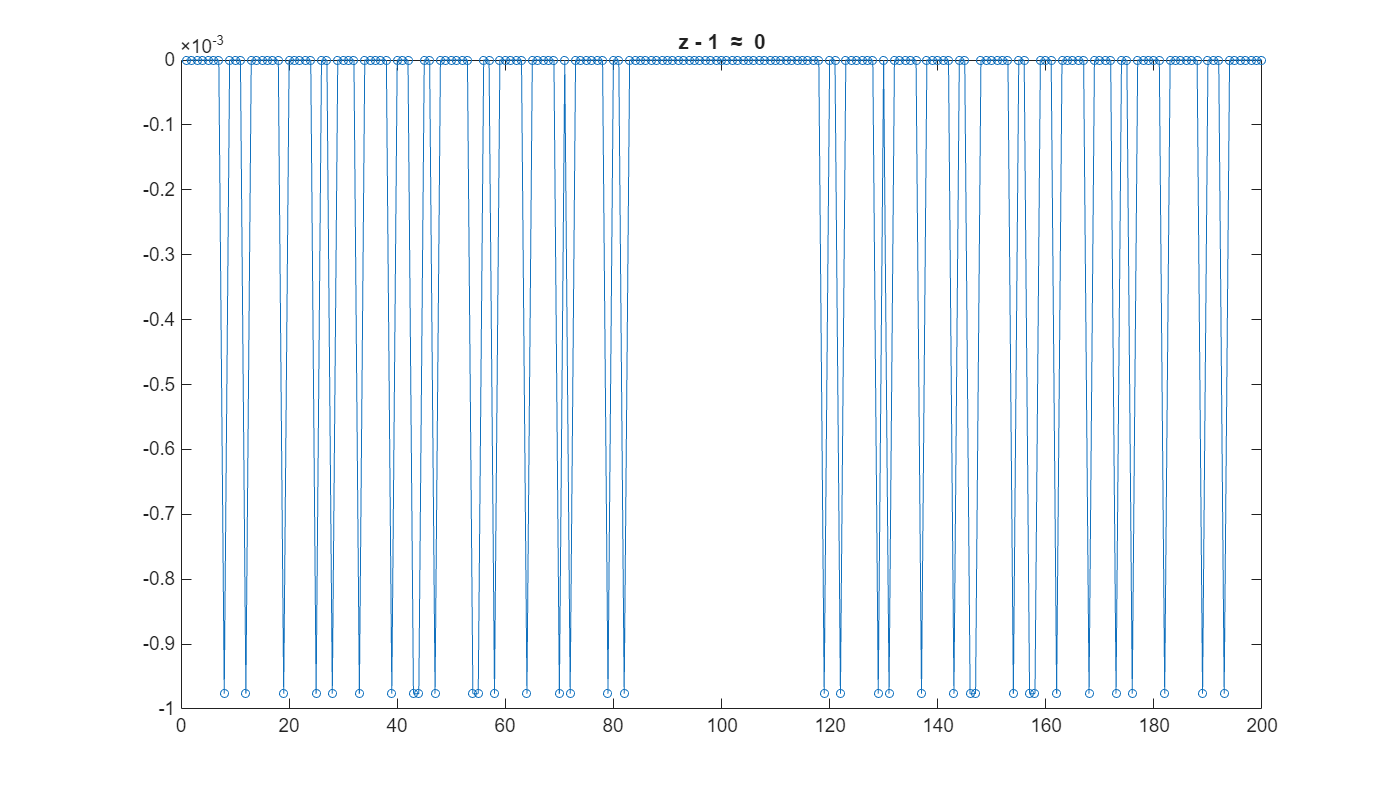## Support평가판 신청